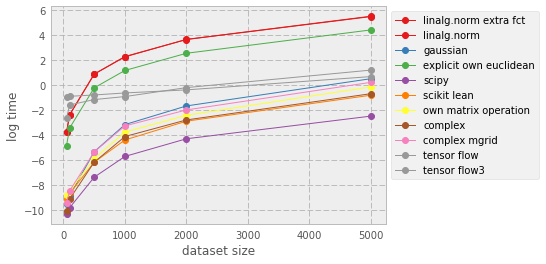## Distance Matrices¶

Calculating distances is a fundamental task in geostatistics. Generally, nearby values are likely to be more similar than pairs of points that are separated by a large distance. This fundamental property is considered in second order based methods as well as in many other flavours of geostatistics, including copulas. Even more, calculating distances is equally important in other disciplines, such as machine learning (see blog randomlydistributed).

This notebook compares a few algorithms that can be used to calculate distances

1. using an euclidean disctance using np.norm
2. same as 1, but with an explicit loop
3. using a Gaussian type expression $[(X-X^T)^2+(Y-Y^T)^2]^{0.5}$
4. a traditional "for" loop
5. using scipy
6. using scikit-learn
7. using a own-written matrix operation
8. using complex numbers
9. using complex numbers with meshgrid
10. using tensorflow
11. using tensorflow version 2

The jupyter notebook is the basis of this post, the underlying python module with all the ways to calculate distance matrices is available here.

This notebook was created with the help of Bo Xiao, Sebastian Gnann, and Thomas Pfaff.

Result: scipy performs best; tensorflow is not bad, it seems to become advantageous for very large data-sets.

All the analysis is based on %timeit for now.

In :
import sys
import numpy as np
import scipy as sp
import sklearn
import matplotlib as mpl
import matplotlib.pyplot as plt
import tensorflow as tf
from py import dist_mat_helpers as dmh

print(sys.executable)
print ("np:       {}".format(np.__version__))
print ("sp:       {}".format(sp.__version__))
print ("sklearn:  {}".format(sp.__version__))
print ("mpl:      {}".format(mpl.__version__))
print ("tf:       {}".format(tf.__version__))

%matplotlib inline

/Users/claushaslauer/anaconda/envs/py36/bin/python
np:       1.11.3
sp:       0.18.1
sklearn:  0.18.1
mpl:      2.0.0
tf:       1.0.0

In :
dataset_sizes = [50, 100, 500, 1000, 2000, 5000]
options_calc_dist = [dmh.calc_distance_bo1_wrapper,       # Bo1a: linalg.norm, explicit loop
#              in extra function (cacl_euclidean distance)
dmh.calc_distance_bo1_2_wrapper,     # Bo1b: linalg.norm, explicit loop
dmh.calc_distance_bo2_wrapper,       # Bo2a: $[(X-X^T)^2+(Y-Y^T)^2]^{0.5}$
dmh.calc_distance_sebastian_wrapper, # Sebastian: own written euclidean function
dmh.calc_distance_scipy,              # SciPy
dmh.calc_distance_sklearn,            # SciKitLearn
dmh.calc_distance_chtp_wrapper,       # via matrix operation
dmh.calc_distance_complex,            # complex
dmh.calc_distance_complex2,           # complex meshgrid
dmh.calc_distance_tf,
dmh.calc_distance_tf3]                 # tensor flow

options_names = ['linalg.norm extra fct',
'linalg.norm',
'gaussian',
'explicit own euclidean',
'scipy',
'scikit lean',
'own matrix operation',
'complex',
'complex mgrid',
'tensor flow',
'tensor flow3']

In :
list_timings = []
for cur_i, cur_option in enumerate(options_calc_dist):
timings = []
print (options_names[cur_i])
for cur_size in dataset_sizes:
res_1 = %timeit -q -o cur_option(cur_size)
timings.append(res_1.best)
list_timings.append(timings)

linalg.norm extra fct
linalg.norm
gaussian
explicit own euclidean
scipy
scikit lean
own matrix operation
complex
complex mgrid
tensor flow
tensor flow3

In :
n_lines = len(options_names)
colors = np.linspace(0, 1, n_lines)
#plt.set_color_cycle([plt.cm.cool(i) for i in ])
for cur_i, cur_name in enumerate(options_names):
plt.plot(dataset_sizes,
np.log(list_timings[cur_i]),
'o-',
label=cur_name,
color=plt.cm.Set1(colors[cur_i]))
plt.xlabel('dataset size')
plt.ylabel('log time')
#plt.legend(loc='upper left')
plt.legend(bbox_to_anchor=(1, 1), loc='upper left', ncol=1)

Out:
<matplotlib.legend.Legend at 0x115f59ba8>© Claus Haslauer. Built using Pelican. Theme by Giulio Fidente on github.